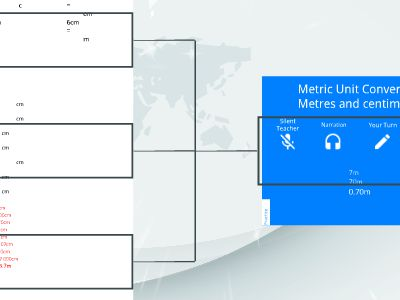Publications: 74 | Followers: 0

## Metres-and-centimetres

Publish on Category: Birds 0

Metric Unit Conversion:Metres and centimetres
SilentTeacher
Narration
Your Turn
IntelligentPractice
Practice
7m70m0.70m
Worked Example
Your Turn
4m = cm60cm = m
@mrbartonmaths
40m = cm6cm = m
1.7m = cm2.70m = cm3.0.70m = cm4.0.7m = cm5.0.07m = cm6.3.07m = cm7.3.7m = cm8.370m = cm9.370cm = m
@mrbartonmaths
1.7m =700cm2.70m =7000cm3.0.70m =70cm4.0.7m =70cm5.0.07m =7cm6.3.07m =307cm7.3.7m =370cm8.370m =37 000cm9.370cm =3.7m
@mrbartonmaths

0

Embed

Share

Upload

Make amazing presentation for free
Metres-and-centimetres